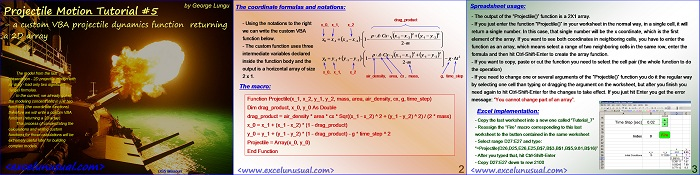# 2D Projectile Motion Tutorial #7

In the this tutorial, after we got most of the trajectory calculation concentrated in just two columns, we will write a custom VBA function (dual output) to replace the spreadsheet computations used.

This process of  starting with very simple models, then refining the calculations and then learning how to write custom functions for those calculations will be extremely useful later for developing more complex models.[sociallocker][/sociallocker]

## Projectile Motion Tutorial #5 – a custom VBA projectile dynamics function returning

by George Lungu

The model from the last
presentation – 2D projectile motion with
air drag – had only two significant
(large) formulas.

In the current, we already got all
the modeling concentrated in just two
functions (the coordinate functions)
therefore we will write a custom VBA
function (returning a 2D array).

This process of concentrating the
calculations and writing custom
functions for those calculations will be
extremely useful later for building
complex models.

<excelunusual.com>

### The coordinate formulas and notations:

drag_product
– Using the notations to the right inside the function body and the output is a horizontal array of size
x_0, x_1, x_2
2 x 1. air_density, area, cx , mass, g, time_step

#### The macro:

Function Projectile (x_1, x_2, y_1, y_2, mass, area, air_density, cx, g, time_step)
Dim drag_product, x_0, y_0 As Double
drag_product = air_density * area * cx * Sqr((x_1 – x_2) ^ 2 + (y_1 – y_2) ^ 2) / (2 * mass)
x_0 = x_1 + (x_1 – x_2) * (1 – drag_product)
y_0 = y_1 + (y_1 – y_2) * (1 – drag_product) – g * time_step ^ 2
Projectile = Array(x_0, y_0)
End Function

<excelunusual.com> 2

### Spreadsheet usage:

– The output of the “Projectile()” function is a 2X1 array.

– If you just enter the function “Projectile()” in your worksheet in the normal way, in a single cell, it will
return a single number. In this case, that single number will be the x coordinate, which is the first
element of the array. If you want to see both coordinates in neighboring cells, you have to enter the
function as an array, which means select a range of two neighboring cells in the same row, enter the
formula and then hit Ctrl-Shift-Enter to create the array function.

– If you want to copy, paste or cut the function you need to select the cell pair (the whole function to do
the operation)

– If you need to change one or several arguments of the “Projectile()” function you do it the regular way
by selecting one cell than typing or dragging the argument on the worksheet, but after you finish you
need again to hit Ctrl-Shift-Enter for the changes to take effect. If you just hit Enter you get the error
message: “You cannot change part of an array”.

### Excel implementation:

– Copy the last worksheet into a new one called “Tutorial_7”

– Reassign the “Fire” macro corresponding to this last
worksheet to the button contained in the same worksheet

– Select range D27:E27 and type: “=Projectile(D26,D25,E26,E25,B\$7,B\$3,B\$1,B\$5,9.81,B\$16)”

– After you typed that, hit Ctrl-Shift-Enter

– Copy D27:E27 down to row 2100

by George Lungu <excelunusual.com>

### 2 Comments

1.Thanks for the comment Mark. Your formulas and assumption are too obvious to mention. Contrary to what you are saying my model was designed for static air and it does take into account the relative speed of the projectile relative to air. To give you the proof, take your formulas and plug in zero wind speed and you shold get the same result. My goal was rather proving the numeric method and not getting into the little details (there are much better free tools out there I believe which have all the options that shooters could use). Had I done that, I would have considered the correct Cx in the first place (sub, trans and supersonic) and the wind on both coordinates, transversal Cx, Coriolis acceleration, Magnusson effect with the rotation of projectile etc etc. The correct Cx has a far larger effect than say, few meters of frontal wind. George

2.I too have been modeling projectile motion in excel but for particulates in an air stream. The way the drag force equation, F_d = 1/2 * rho_air * C_d * A * V^2, is used in your models, it only takes into account the velocity of the projectile itself. The velocity in this equation actually refers to the relative velocity of the projectile in the medium it is traveling in. For example, if a projectile is fired horizontally at 5 m/s into a tailwind also at 5 m/s, then the V in F_d in the X direction is zero. The projectile would only experience drag forces in the Y direction as it falls. The corrected equation for drag is F_d = 1/2 * rho_air * C_d * A_projectile * (V_projectile – V_air)^2. This small change adds a lot of accuracy to your model.